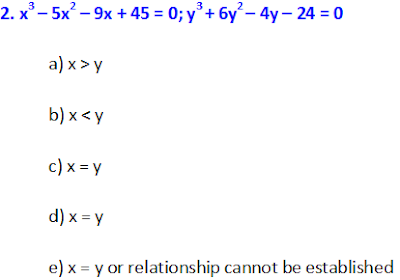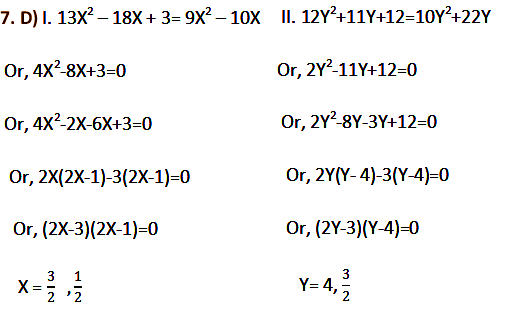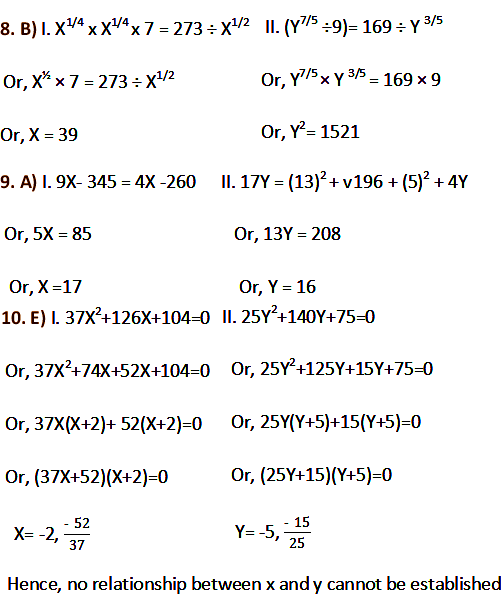## Quantitative Aptitude Quadratic Equations Questions (14 – 09 – 2017)

Quantitative Aptitude Quadratic Equations Questions (14 – 09 – 2017)
Directions (1-5): In each of these questions, two or three equations are given. You have to solve both the equations and give answer
1. 6x + 9y = 23; 4x + 7y = 42; x + z = 42
a) x < y = z
b) x < y < z
c) x > y > z
d) x ≤ y < z
e) x = y = z or relation cannot be established3. √(x + 5) + √1024 = √1369;   √y(y-5) = √1764 – √1296
a) x > y
b) x < y
c) x ≥ y
d) x ≤ y
e) x = y or relation cannot be established
4. (3x – 2)/y = (3x + 6)/(y + 16)
(x + 2)/(y + 4) = (x + 5)/(y + 10)
a) x > y
b) x < y
c) x ≥ y
d) x ≤ y
e) x = y or relation cannot be established
5. (x – 2)(x + 1) = (x – 1)(x + 3)
(y + 3)(y – 2) = (y + 1)(y + 2)
a) x > y
b) x < y
c) x ≥ y
d) x ≤ y
e) x = y or relation cannot be established
Directions (6 – 15): In the following questions two equations numbered I and II are given. You have to solve both the equations and Give answer
a) If X > Y
b) If X ≥ Y
c) If X< Y
d) If X≤ Y
e) X=Y or Relationship cannot be established.Solutions:
1. B) Solving 1st and 2nd equations, we get x = 217/6 ≈ -36; y = 80/3 ≈ 26
substituting in 3rd eqn, z = 78
X < Y < Z
3. A) √(x + 5) + 32 = 37; √(x + 5) = 5;
Squaring on both sides, (x + 5) = 25
X = 20;
√y(y-5) = 42 – 36; √y(y-5) = 6;
Squaring on both sides, y(y-5) = 36;
Y2 – 5y – 36 = 0; y = +9, -4;
X > Y
4. B) (3x – 2)/y = (3x + 6)/(y + 16)
y + 16(3x – 2) = y (3x + 16)
48x – 8y = 32
6x – y = 4 —(1)
(x + 2)/(y + 4) = (x + 5)/(y + 10)
(x + 2)(y + 10) = (x + 5)(y + 4)
2x = y —(2)
Substitute (2) in (1),
x = 1, y = 2
5. A) (x – 2)(x + 1) = (x – 1)(x + 3)
X2 + x – 2x – 2 = x2 + 3x – x – 3
x = 1/3
(y + 3)(y – 2) = (y +1)(y + 2)
Y2 –2y + 3y – 6 = y2 + 2y
y = – 4
6. C) I. (257)1/4 X + (217)1/3 =0
Or, 4.004 X+6.01 = 0
Or, 4.004X= - 6.01
X = - 1.5
II. √1100 Y + √1295 = 0
Or, 33.17 Y+ 35.99 = 0
Or,  Y= - 1.111. B) x = -3/4, -1/2; y = -3, -4/5 Therefore x > y
12. D) x = -2/3, -1/3; y = -7/4, -2/3 Therefore x ≥ y
13. D) x = 1/5, ½; y = 1/7, 1/5 Therefore x ≥ y
14. B) I. x – 7√2 x + 24 = 0 => (√x - 3√2)( √x - 4√2) = 0;
If √x = 3√2 => x = 18 and if √x = 4√2 => x = 32
II. y – 5√2 y + 12 = 0 => (√y - 2√2)( √y - 3√2) = 0;
If √y = 2√2 => y = 8 and if √y = 3√2 => y = 18
Therefore x ≥ y